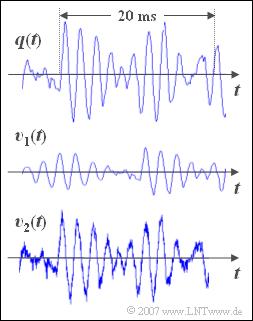# Exercise 1.1: Music SignalsMusic signals, original,
noisy and/or distorted?

On the right you see a  $\text{30 ms}$  long section of a music signal  $$q(t)$$.  It is the piece  "For Elise"  by Ludwig van Beethoven.

• Underneath are drawn two sink signals  $$v_1(t)$$  and  $$v_2(t)$$, which were recorded after the transmission of the music signal  $$q(t)$$  over two different channels.
• The following operating elements allow you to listen to the first fourteen seconds of each of the three audio signals  $$q(t)$$,  $$v_1(t)$$  and  $$v_2(t)$$.

Original signal  $$q(t)$$:

Sink signal  $$v_1(t)$$:

Sink signal  $$v_2(t)$$:

Notes:

### Questions

1

Estimate the signal frequency of  $$q(t)$$  in the displayed section.

 The signal frequency is approximately  $$f = 250\,\text{Hz}$$. The signal frequency is approximately  $$f = 500\,\text{Hz}$$. The signal frequency is about  $$f = 1\,\text{kHz}$$.

2

Which statements are true for the signal  $$v_1(t)$$ ?

 The signal  $$v_1(t)$$  is undistorted compared to  $$q(t)$$. The signal  $$v_1(t)$$  shows distortions compared to  $$q(t)$$ . The signal  $$v_1(t)$$  is noisy compared to  $$q(t)$$ .

3

Which statements are true for the signal  $$v_2(t)$$ ?

 The signal  $$v_2(t)$$  is undistorted compared to  $$q(t)$$ . The signal  $$v_2(t)$$  shows distortions compared to  $$q(t)$$ . The signal  $$v_2(t)$$  is noisy compared to  $$q(t)$$ .

4

One of the signals is undistorted and not noisy compared to the original   $$q(t)$$ .
Estimate the attenuation factor and the delay time for this.

 $$\alpha \ = \$$ $$\tau \ = \$$ $\ \text{ms}$

### Solution

#### Solution

(1)  Correct is the solution 2:

• In the marked range of  $20$  milliseconds approx.  $10$  oscillations can be detected.
• From this the result  follows approximately for the signal frequency  $f = {10}/(20 \,\text{ms}) = 500 \,\text{Hz}$.

(2)  Correct is the solution 1:

• The signal  $$v_1(t)$$  is undistorted compared to the original signal $$q(t)$$.  The following applies:   $v_1(t)=\alpha \cdot q(t-\tau)$.
• An attenuation  $$\alpha$$  and a delay  $$\tau$$  do not cause distortion, but the signal is then only quieter and delayed in time, compared to the original.

(3)  Correct are the solutions 1 and 3:

• One can recognize additive noise both in the displayed signal  $$v_2(t)$$  and in the audio signal    ⇒   solution 3.
• The signal-to-noise ratio is approx.  $\text{30 dB}$;  but this cannot be seen from this representation.
• Correct is also the solution 1:   Without this noise component  $$v_2(t)$$  would be identical with  $$q(t)$$.

(4)  The signal  $$v_1(t)$$  is identical in form to the original signal  $$q(t)$$  and differs from it only

• by the attenuation factor  $\alpha = \underline{\text{0.3}}$   $($this corresponds to about  $\text{–10 dB)}$
• and the delay  $\tau = \underline{10\,\text{ms}}$.